# Catalan Solids Covered with Matching Polygons2021/04/18

Beside the Platonic solids the Catalan solids are convex solids with congruent faces and fixed dihedral angles. We can use polygons with colored sides to cover the solids so that same colored sides match at the edges of the solid. If the set of polygons is large enough, this is no problem. Therefore I looked for sets with special properties, so that the number of pieces in the set is equal to the number of faces of a given Catalan solid. In most cases it was easy for my computer program to get a solution, only in case of the pentagonal hexecontahedron a solution wasn't found immediately.

Because of the fixed dihedral angles you can print the congruent polygons with fixed bevels and then glue all of them to get the Catalan solid.

It's also possible to add kind of folds and gaps at these folds to connect the polygons by U-clips. The positions for the gaps are: center (0), right from center (1), left from center (-1), far right from center (2) and far left from center (-2). A gap x then matches a gap -x. At the virtual objects the center positions are always red marked, left and right positions are green marked and the more distant positions are blue marked. Since the sides of the polygons are of different length, equal positions divide the sides by fixed ratios.

Because there are dipyramids and antidipyramids with congruent faces and fixed dihedral angles I also had a look at some of these pyramids.

Click the pictures in the table to see examples of covered Catalan solids and the used sets. If you click these examples you can also see virtual models of them.

## Triakis Tetrahedron

two-sided isosceles triangles,
3 possible colors,
2 sides same colored,
third side differently colored.
(3*3*2+3*2)/2=12 piecestwo-sided isosceles triangles,
4 possible positions for marks,
all positions different,
4*3*2/2=12 pieces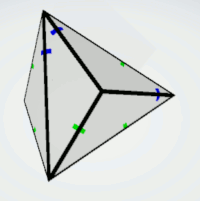one-sided isosceles triangles,
3 possible colors,
at the base only two colors,
at the legs different colors,
2*3*2=12 pieces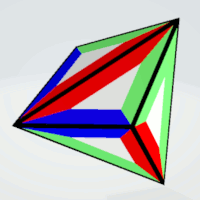one-sided isosceles triangles,
3 possible positons for marks,
at the base only two positions,
at the legs different positions,
2*3*2=12 pieces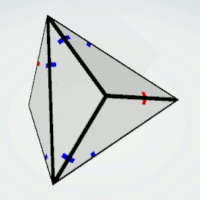This is a real solid where the marks are replaced by gaps, and the triangles are connected at these points.## Triakis Octahedron

one-sided isosceles triangles,
4 possible colors,
all sides differently colored,
4*3*2=24 piecesone-sided isosceles triangles,
4 possible positions for marks,
all positions are different,
4*3*2=24piecesone-sided isosceles triangles,
3 possible colors,
no same colored pieces,
3^3-3=24 piecesone-sided isosceles triangles,
3 possible positons for marks,
not all positions are equal,
3^3-3=24 pieces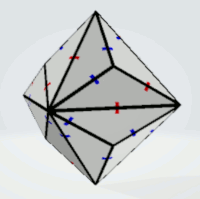## Triakis Icosahedron

one-sided isosceles triangles,
5 possible colors,
all sides differently colored,
5*4*3=60 pieces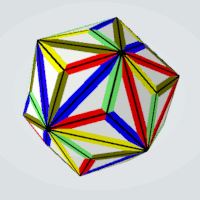one-sided isosceles triangles,
5 possible positions for marks,
all positions are different,
5*4*3=60 piecesone-sided isosceles triangles,
4 possible colors,
no same colored pieces,
4^3-4=60 pieces
No solution, since 60*3/4=5 is odd one-sided isosceles triangles,
4 possible positons for marks,
not all positions are equal,
4^3-4=60 pieces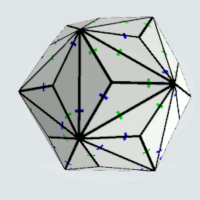This is a real solid where the marks are replaced by gaps, and the triangles are connected at these points.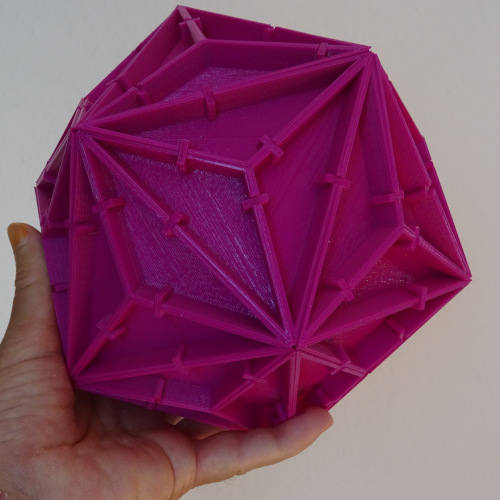## Tetrakis Hexahedron

one-sided isosceles triangles,
4 possible colors,
all sides differently colored,
4*3*2=24 pieces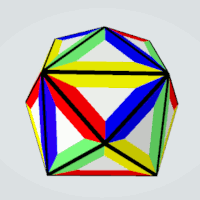one-sided isosceles triangles,
4 possible positions for marks,
all positions are different,
4*3*2=24pieces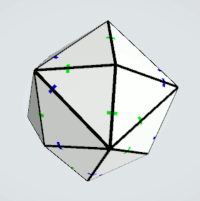one-sided isosceles triangles,
3 possible colors,
no same colored pieces,
3^3-3=24 pieces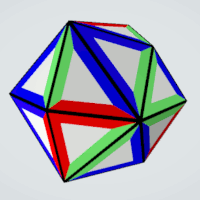one-sided isosceles triangles,
3 possible positons for marks,
not all positions are equal,
3^3-3=24 pieces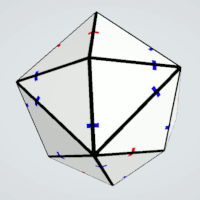## Pentakis Dodecahedron

one-sided isosceles triangles,
5 possible colors,
all sides differently colored,
5*4*3=60 pieces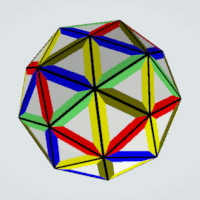one-sided isosceles triangles,
5 possible positions for marks,
all positions are different,
5*4*3=60 piecesone-sided isosceles triangles,
4 possible colors,
no same colored pieces,
4^3-4=60 pieces
No solution, since 60*3/4=5 is odd one-sided isosceles triangles,
4 possible positons for marks,
not all positions are equal,
4^3-4=60 pieces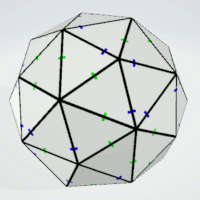This is a real solid where the marks are replaced by gaps, and the triangles are connected at these points.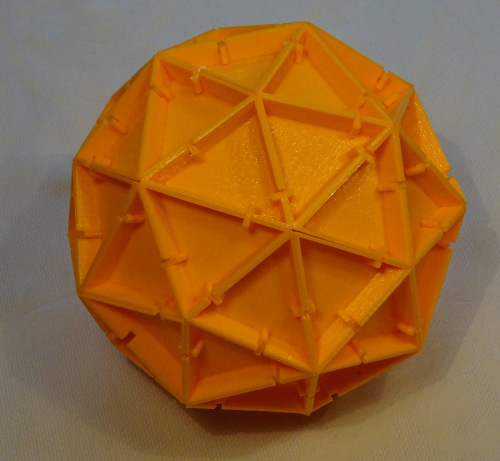## Hexakis Octahedron

Since the 48 congruent faces of the hexakis octahedronare are scalene triangles we have 24 pairs of mirror pieces. For each coloring of one triangle there is a reflected coloring of the mirror piece.

one-sided scalene triangles,
4 possible colors,
all sides differently colored,
2*(4*3*2)=48 pieces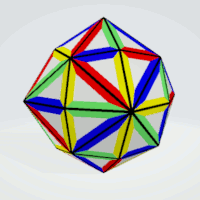one-sided scalene triangles,
4 possible positions for marks,
all positions are different,
2*(4*3*2)=48 pieces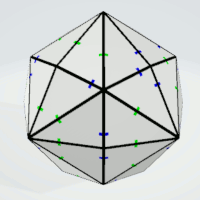one-sided scalene triangles,
3 possible colors,
no same colored pieces,
2*(3^3-3)=48 pieces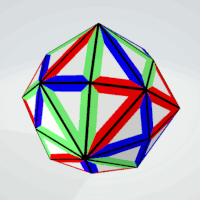one-sided scalene triangles,
3 possible positons for marks,
not all positions are equal,
2*(3^3-3)=48 pieces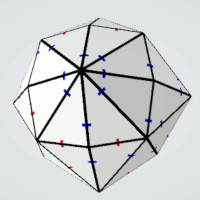Following a computer solution sets of six triangles each were arranged getting eight larger kind of triangles. These parts can be combined to get the whole construction.This is a real solid where the marks are replaced by gaps, and the triangles are connected at these points.## Hexakis Icosahedron

Since the 120 congruent faces of the hexakis icosahedron are scalene triangles we have 60 pairs of mirror pieces. For each coloring of one triangle there is a reflected coloring of the mirror piece.

one-sided scalene triangles,
5 possible colors,
all sides differently colored,
2*(5*4*3)=120 pieces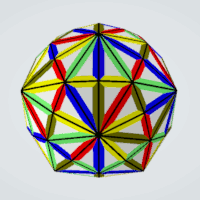one-sided scalene triangles,
5 possible positions for marks,
all positions are different,
2*(5*4*3)=120 pieces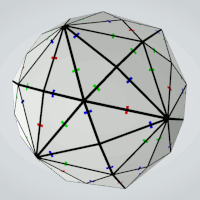one-sided scalene triangles,
4 possible colors,
no same colored pieces,
2*(4^4-4)=120 pieces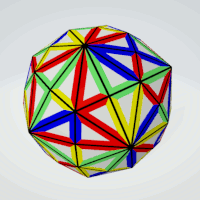one-sided scalene triangles,
4 possible positons for marks,
not all positions are equal,
2*(4^4-4)=120 pieces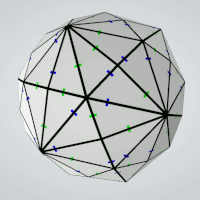## Dipyramids

one-sided isosceles triangles,
3 possible colors,
2 sides same colored,
one side with another color
3*3*2=18 pieces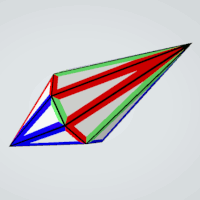one-sided isosceles triangles,
3 possible positions for marks,
2 marks at same positions,
one mark at another position,
3*3*2=18 piecestwo-sided isosceles triangles,
3 possible colors,
(3^3+3^2)/2=18 pieces,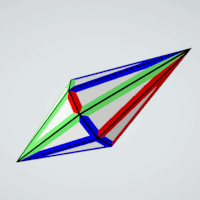one-sided isosceles triangles,
2 possible positons for marks at the base,
3 possible positions for marks at the legs,
marked positions at the legs must be different,
2*3*2=12 pieces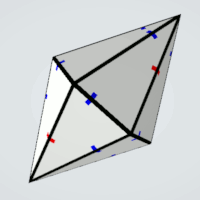two-sided isosceles triangles,
3 possible colors,
2 sides same colored,
one side with another color,
(3*3*2+3*2)/2=12 pieces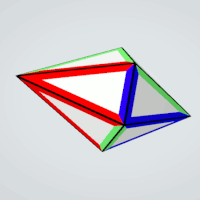one-sided isosceles triangles,
3 possible positons for marks,
not all positions are equal,
3^3-2=24 pieces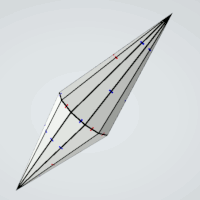## Rhombic Dodecahedron

one-sided rhombs,
3 possible colors,
exactly 3 sides are same colored
2*(3*2)=12 pieces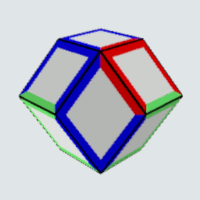one-sided rhombs,
3 possible positions for marks,
exactly three side with same positions of marks,
2*(3*2)=12 pieces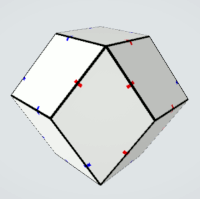This is a real solid where the marks are replaced by gaps, and the kites are connected at these points.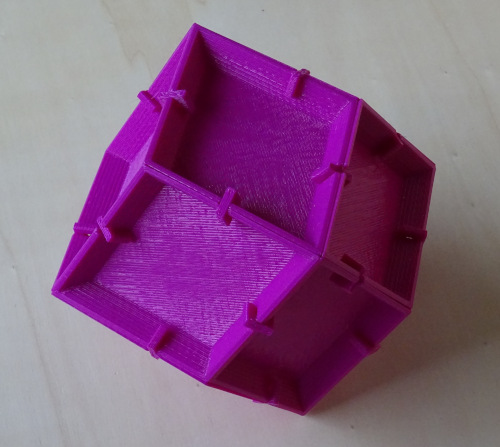## Rhombic Triacontahedron

one-sided rhombs,
3 possible colors,
no more than two same colored sides,
4*3*2/2+2*3+2*3*2=30 pieces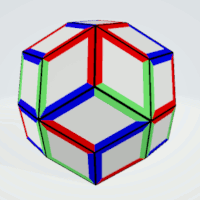one-sided rhombs,
3 possible positions for marks,
no more than two equal positions of marks,
4*3*2/2+2*3+2*3*2=30=30 piecesone-sided rhombs,
6 possible colors,
opposite sides same colored,
two colors used,
6*5=30 pieces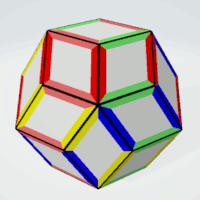two-sided rhombs,
5 possible colors,
all sides differently colored,
=30 piecesAfter I had glued a rhombic triacontahedron I stuck iron foil on the faces. Then printed colored rhombs were stuck on magnetic foil to put them onto the solid.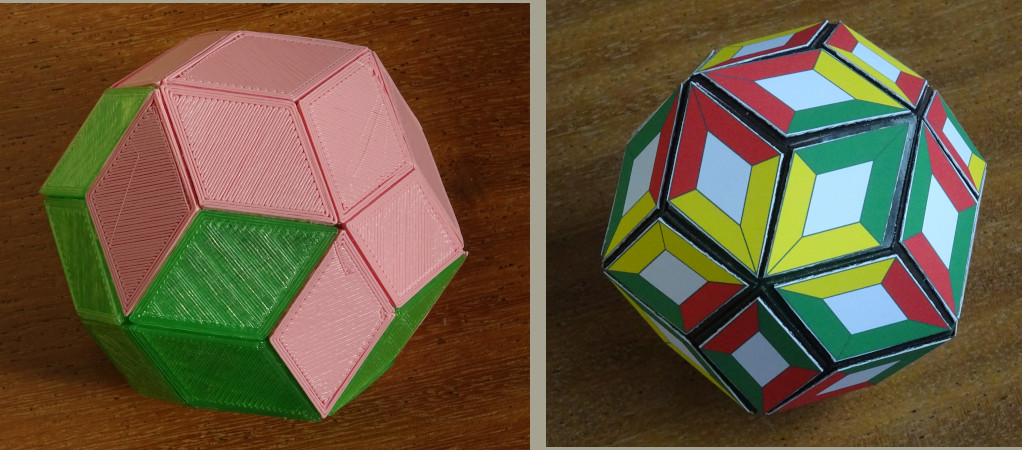The rhombs can also be used to fill a parallelogram of size 5x6.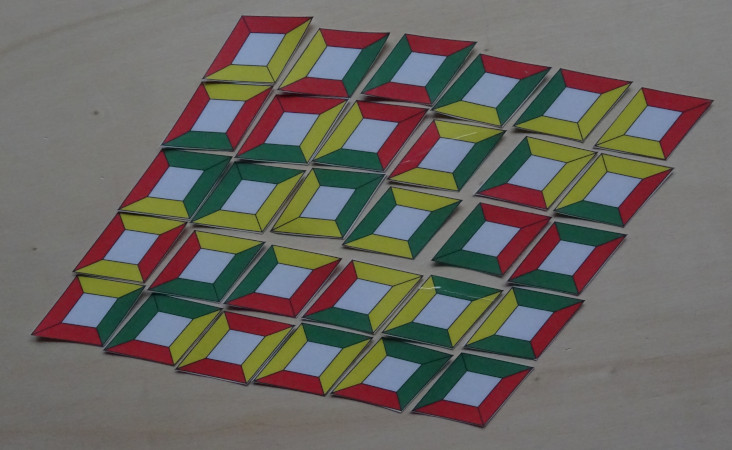## Deltoidal Icositetrahedron

one-sided kites,
4 possible colors,
all sides differently colored,
4*3*2*1=24 pieces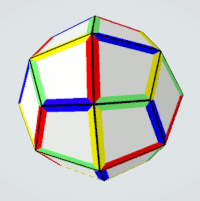one-sided kites,
4 possible positions for marks,
all positions are different,
4*3*2*1=24 pieces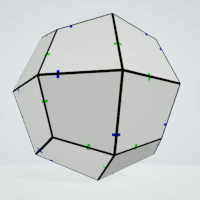This is a real solid where the marks are replaced by gaps, and the kites are connected at these points.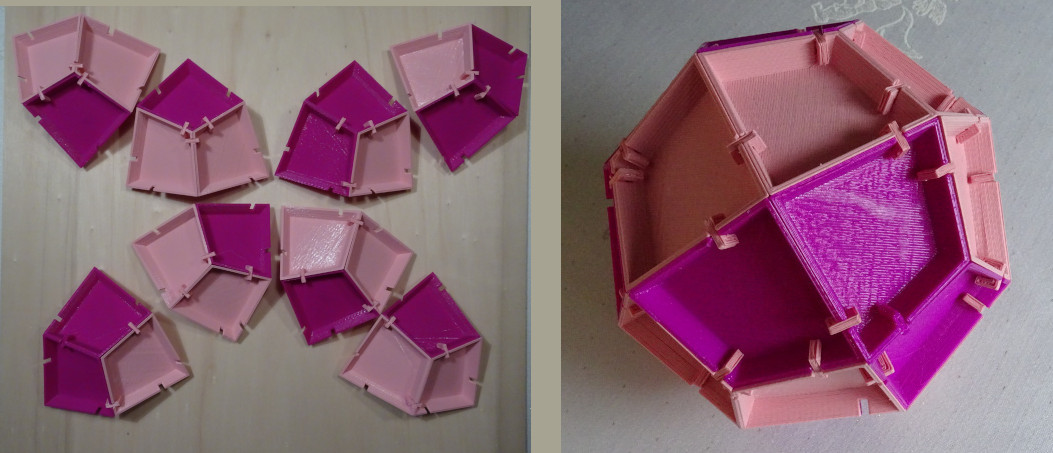## Deltoidal Hexecontahedron

one-sided kites,
5 possible colors,
two pairs of same colored sides,
3*(5*4)=60 pieces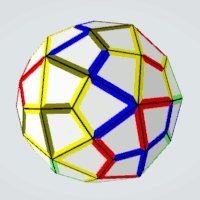one-sided kites,
5 possible positions for marks,
two pairs of sides with equal positions,
3*(5*4)=60 pieces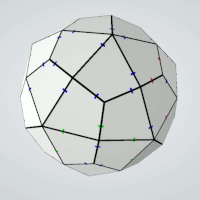## Antidipyramid

one-sided kites,
2 possible colors,
2^4=16 pieces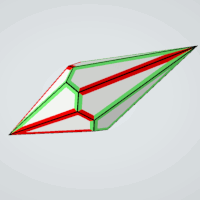one-sided kites,
2 possible positions for marks,
2^4=16 pieces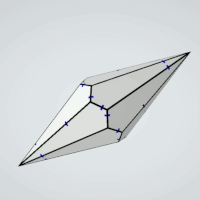one-sided kites,
2 possible colors,
no same colored pieces,
2^4-2=14 pieces
No solution, because 14/2=7 is odd one-sided kites,
2 possible positions for marks,
not all marks at equal positions,
2^4-2=14 pieces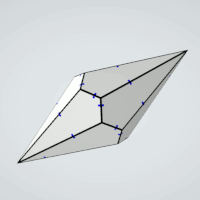two-sided kites,
2 possible colors,
(2^4+2^2)/2=10 piecestwo-sided kites,
2 possible positions for marks,
(2^4+2^2)/2=10 pieces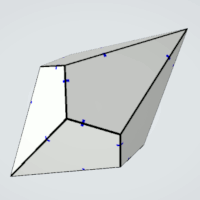## Pentagonal Icositetrahedron

one-sided pentagonss,
3 possible colors,
2 colors for the long sides,
3 different colors for the short sides,
2^2*3*2*1=24 pieces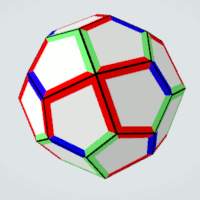one-sided pentagons,
3 possible positions for marks,
2 positions for marks at the long sides,
3 different positions for marks at the short sides,
2^2*3*2*1=24 pieces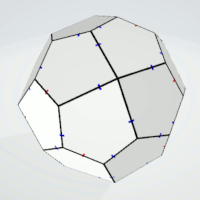This is a real solid where the marks are replaced by gaps, and the pentagons are connected at these points.## Pentagonal Hexecontahedron

one-sided pentagons,
3 possible colors,
one color for 3 sides another color for 2 sides,
(5*4/2)*3*2=60 pieces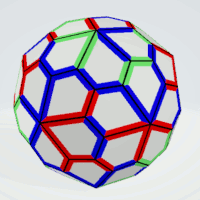one-sided pentagons,
3 possible positions for marks,
3 sides with equal positions 2 sides with another position,
(5*4/2)*3*2=60 pieces